# Step 1. Obtain a 600 mL beaker, andadd 300 mL of water. Step 2. Add 30...

Step 1. Obtain a 600 mL beaker, andadd 300 mL of water.

Step 2. Add 30 mL of HCl (2M) to the beaker, and stir.

Step 3. Add 10 mg of magnesium metal to the beaker.

Step 4. Allow thehydrogen gas to evolve. All of the magnesium should be consumed.

Step 5. Record the amount of hydrogen gas that has evolved using the chemical property dialog.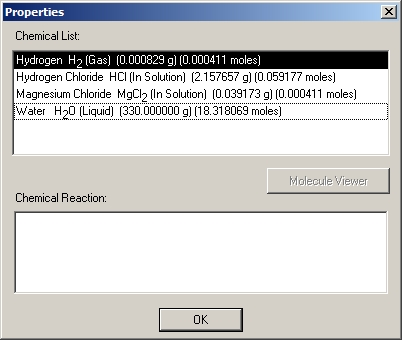questions:

In your lab report, include the following information in the Discussion section of your report:

Record the moles of hydrogen gas that has evolved. Calculate the atomic weight of magnesium as follows:
• atomic weight of Mg (in grams) = weight (in grams) of Mg consumed / moles ofH2 evolved
Discuss the stoichiometry of the chemical reaction that you are studying: Mg + 2HCl --> H2 + Mg2+ (aq) + 2Cl- (aq) How does the information in the balanced chemical equation above help you determine the atomic weight of magnesium? Discuss how the calculated atomic weight of the magnesium compare to information found on the periodic table of elements. <Explain, analyze and interpret what you observed. Draw conclusions from what you know. This is also where you show the reader that you understand the significance or meaning of the results. Refer to iLab instructions for items to include in your discussion.> < Within a few sentences, provide a concluding statement about the results of your laboratory>

Given reactions is

Mg + 2HCl --> H2 + Mg2+ (aq) + 2Cl-(aq)Hydrogen H2 (g) (0.060480g=0.030000moles

Magnesium Mg (solid) (9.270850g = 0.381438 moles

Magnesium Chloride MgCI2 (in solution)2.856300g=(0.030000moles)

Water H2O (liquid) (330g) =(18.318069 moles)

Moles of hydrogen evolved = 0.0300 moles

a) Atomic weight of Mg = weight of Mg consumed / moles of H2

= (10g - 9.27085 g ) / 0.0300 mol

=24.305 g/mol

b)

Number of molecules of H2that were produced in the reaction

= moles of H2* Avogadro's number

No. of H2 molecules= \(1.806 * 10^{22}\)

c)

Number of atoms of Magnesium that were involved in the reaction

= moles of Mg * Avagadro's number

. atoms.

= \(1.8069 * 10^{23}\) atoms.

the reaction is given as:Mg + 2HCl --> H2 + Mg2+ (aq) + 2Cl-.now,we have the amount of H2 evolved:0.4166milimol,as the reacvtion suggests that 1mol Mg:1 mol H2,so,the atomic weight of Mg; (10/0.4166)= 24.0038 gm/mol

#### Earn Coin

Coins can be redeemed for fabulous gifts.

Similar Homework Help Questions
• ### tration : Use a small beaker. I00-mL or 150-ml beaker and fill about ½ full of...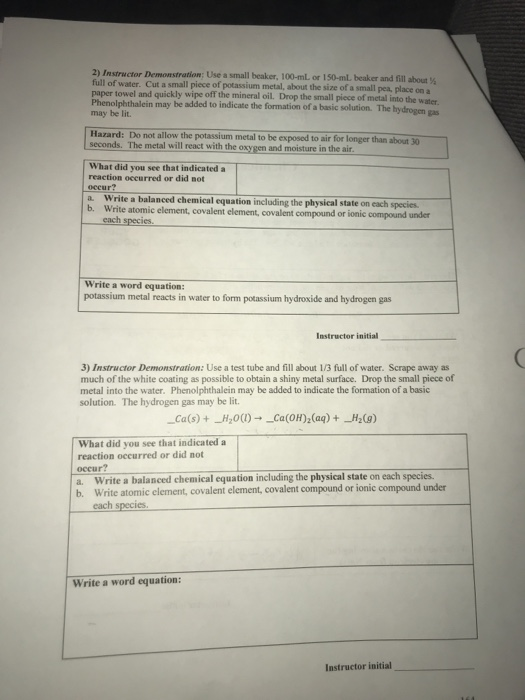tration : Use a small beaker. I00-mL or 150-ml beaker and fill about ½ full of water. Cut a small piece of potassium metal, about the size of a small pea, place on a paper towel and quickly wipe off the mineral oil. Drop the small piece of metal into the Phenolphthalein may be added to indicate the formation of a basic solution. The may be lit. gas Hazard: Do not allow the potassium metal to be exposed to air...

• ### Mass of Magnesium strip Use the length of your magnesium strip and the mass per m...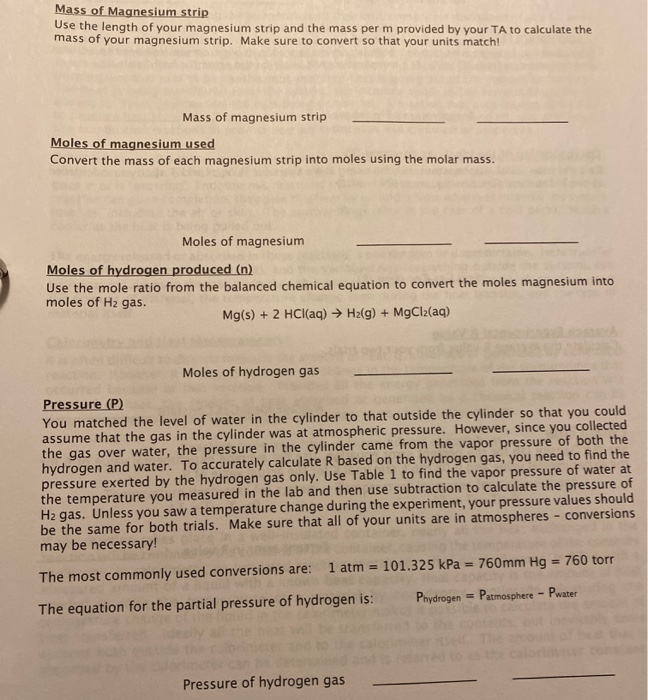Mass of Magnesium strip Use the length of your magnesium strip and the mass per m provided by your TA to calculate the mass of your magnesium strip. Make sure to convert so that your units match! Mass of magnesium strip Moles of magnesium used Convert the mass of each magnesium strip into moles using the molar mass. Moles of magnesium Moles of hydrogen produced (n) Use the mole ratio from the balanced chemical equation to convert the moles magnesium...

• ### Balance the equation for the reaction observed: _ Cu(s) + HNO3(aq) → Cu(NO3)2(aq) +_ NO2(g) + H2O(1) 3. Add 40 mL...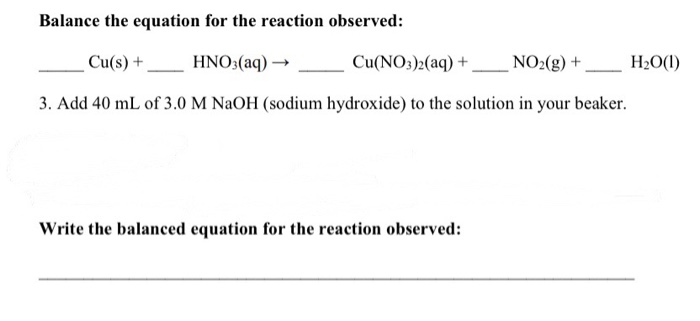Balance the equation for the reaction observed: _ Cu(s) + HNO3(aq) → Cu(NO3)2(aq) +_ NO2(g) + H2O(1) 3. Add 40 mL of 3.0 M NaOH (sodium hydroxide) to the solution in your beaker. Write the balanced equation for the reaction observed: 4. Add 2-3 boiling chips to your beaker and carefully heat the solution, while stirring with a stirring rod, just to the boiling point. (Do NOT boil. Have a low flame and keep the beaker well above the flame.)...

• ### How many moles of H2O2 were consumed? Can you make comments during each step of your...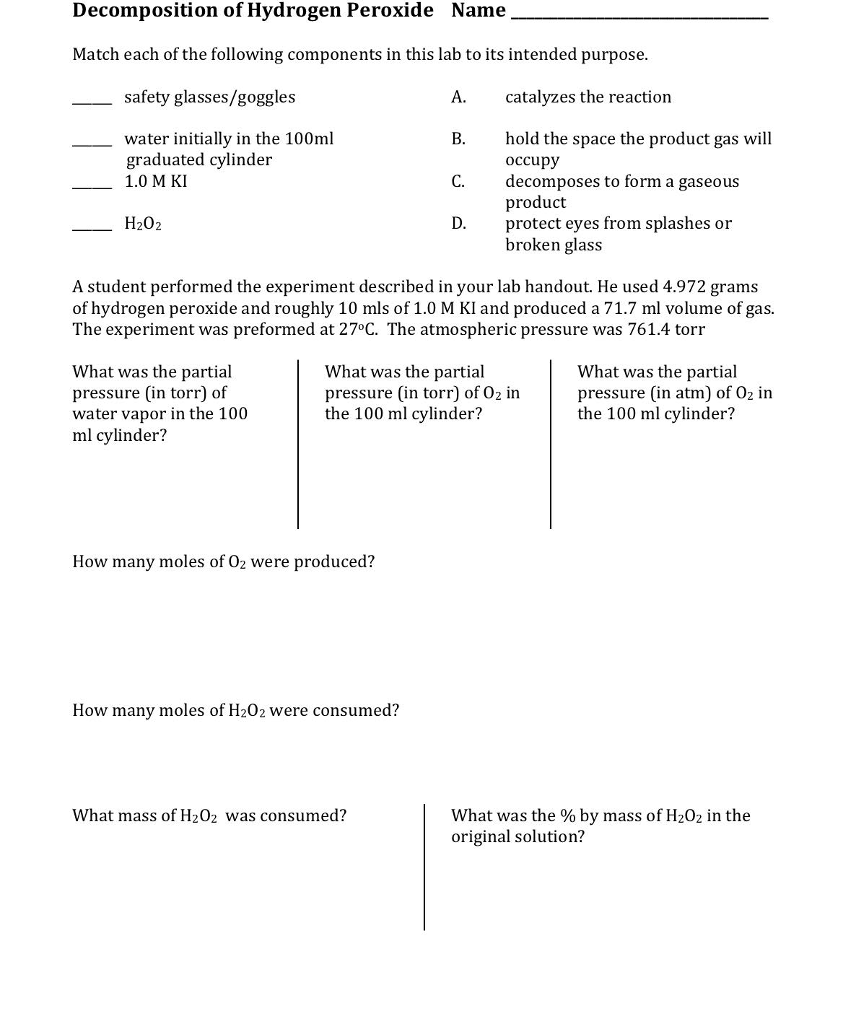How many moles of H2O2 were consumed? Can you make comments during each step of your solution? Decomposition of Hydrogen Peroxide Name Match each of the following components in this lab to its intended purpose safety glasses/goggles catalyzes the reaction water initially in the 100ml graduated cylinder 1.0 M KI hold the space the product gas will occupy decomposes to form a gaseous product protect eyes from splashes or broken glass C. H202 A student performed the experiment described in...

• ### Please answer all questions in both worksheets! Thank you! 1. What is the ideal gas law?...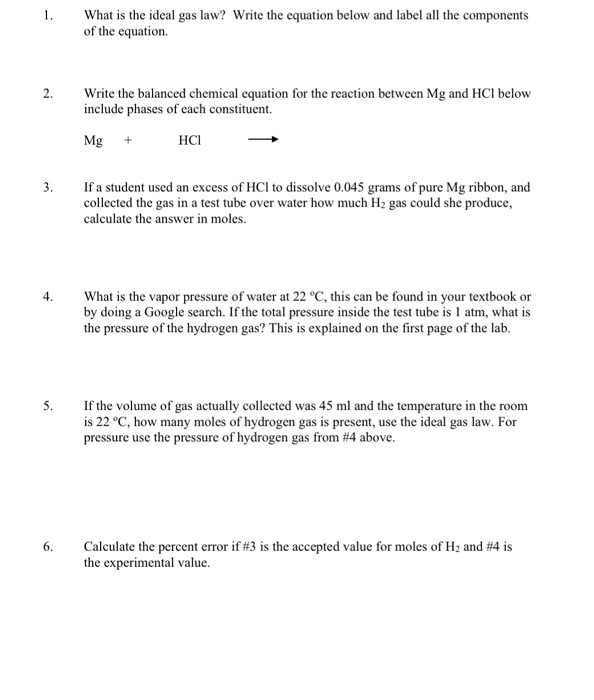Please answer all questions in both worksheets! Thank you! 1. What is the ideal gas law? Write the equation below and label all the components of the equation. 2. Write the balanced chemical equation for the reaction between Mg and HCI below include phases of each constituent. Mg + HCI 3. If a student used an excess of HCl to dissolve 0.045 grams of pure Mg ribbon, and collected the gas in a test tube over water how much H2...

• ### Please help with the post lab and the pre lab (except for obviously #1 on the...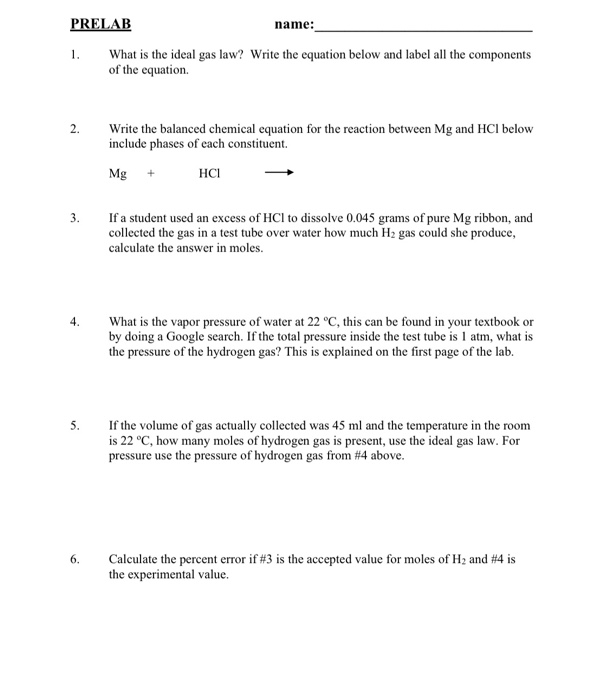Please help with the post lab and the pre lab (except for obviously #1 on the post lab) thank you! PRELAB name: What is the ideal gas law? Write the equation below and label all the components of the equation. Write the balanced chemical equation for the reaction between Mg and HCl below include phases of each constituent. Mg + HCI 3. If a student used an excess of HCl to dissolve 0.045 grams of pure Mg ribbon, and collected...

• ### EXPERIMENTAL PROCEDURE 1. Using a 50.0 mL graduated cylinder, obtain 40.0 mL of the unknown water...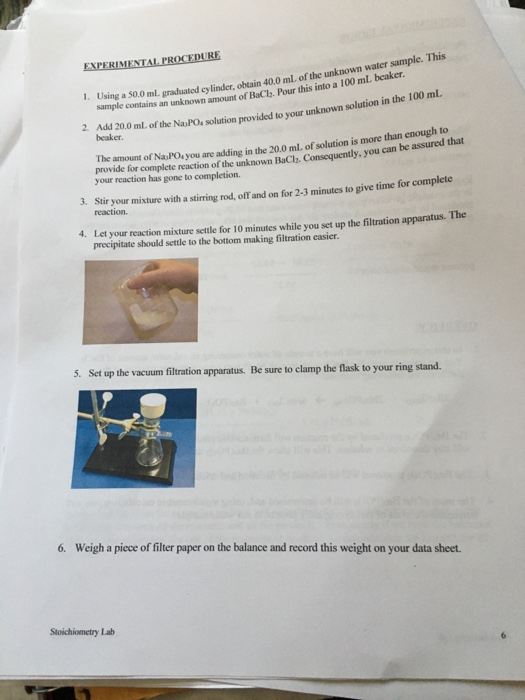EXPERIMENTAL PROCEDURE 1. Using a 50.0 mL graduated cylinder, obtain 40.0 mL of the unknown water sample. This ample contains an unknown amount of BaCh, Pour this into a 100 mL beaker 2. Add 20.0 ml. of the NasPO solution provided to your unknown solution in the 100 ml, beaker The amount of NaPOs you are adding in the 20.0 ml, of solution is more than enough to provide for complete reaction of the unknown BaClh, Consequently, you can be...

• ### help answer all the ones you can. PRELAB name: Jasmine Cancios 1. What is the ideal...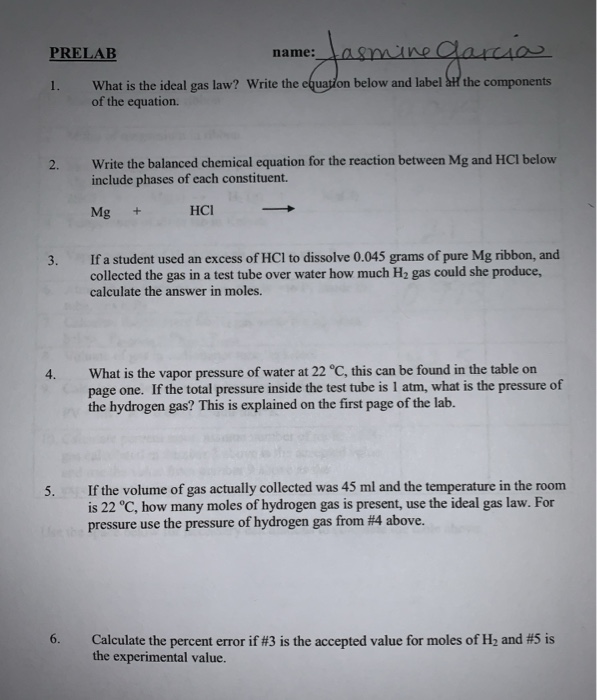help answer all the ones you can. PRELAB name: Jasmine Cancios 1. What is the ideal gas law? Write the equation below and label & the components of the equation. Write the balanced chemical equation for the reaction between Mg and HCl below include phases of each constituent. Mg + НСІ If a student used an excess of HCl to dissolve 0.045 grams of pure Mg ribbon, and collected the gas in a test tube over water how much H,...

• ### i uploaded everything that was given. Where would I find the experiemental values? Complete the calcula...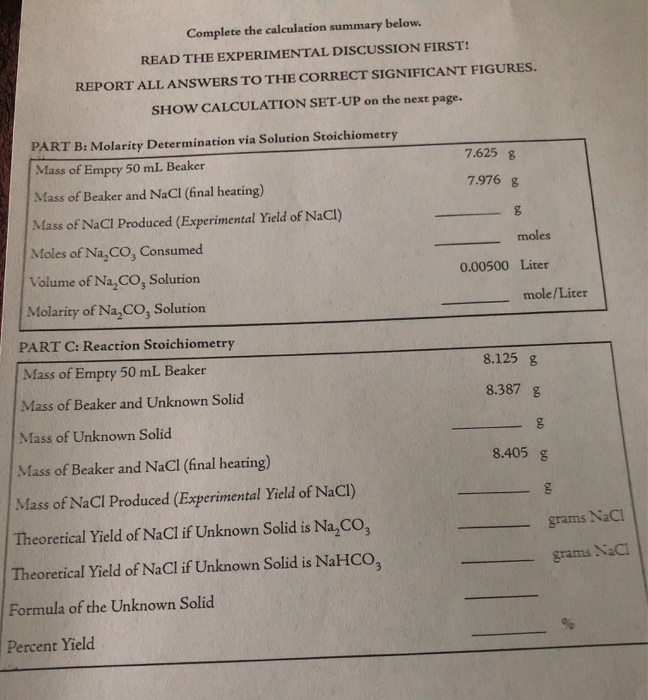i uploaded everything that was given. Where would I find the experiemental values? Complete the calculation summary below. READ THE EXPERIMENTAL DISCUSSION FIRST! REPORT ALL ANSWERS TO THE CORRECT SIGNIFICANT FIGURES. SHOW CALCULATION SET-UP on the next page. PART B: Molarity Determination via Solution Stoichiometry 7.625 g Mass of Empty 50 mL Beaker 7.976 g Mass of Beaker and NaCl (final heating) Mass of NaCl Produced (Experimental Yield of NaCl) moles Moles of Na,CO, Consumed 0.00500 Liter Volume of Na,CO,...

• ### Hello, these are part of one question, please show work. Data Sheet Chemical Reactions 2. Obtain a...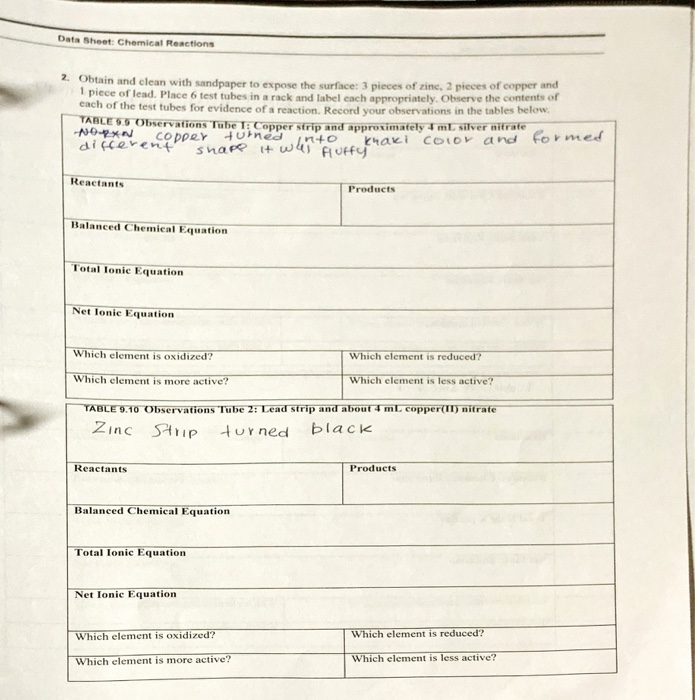Hello, these are part of one question, please show work. Data Sheet Chemical Reactions 2. Obtain and clean with sandpaper to expose the surface: 3 pieces of zine, 2 pieces of copper and 1 piece of lead. Place 6 test tubes in a rack and label each appropriately. Observe the contents of each of the test tubes for evidence of a reaction. Record your observations in the tables below TABLE 55 Observations Tube I: Copper strip and approximately 1 mL...